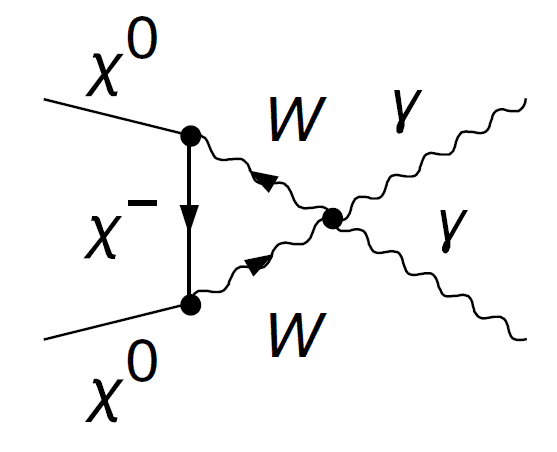# Computing amplitude for divergent loop diagrams?

• A
• Ramtin123

#### Ramtin123

I am trying to compute the cross-section for the diagram below with a divergent triangle loop:where ##X^0## and ##X^-## are some fermions with zero and negative charge respectively. I am interested in low energy limits, so you can consider W-propagator as ##\frac {i\eta_{\mu\nu}} {M_w^2}##.

When computing the amplitude, you end of with an integration of the form:

$$\int \frac {k_\mu \gamma^\mu +m_-} {k^2 -m_-^2} \frac {d^4 k} {(2\pi)^4}$$

where ##m_-## is mass of ##X^-##.

Any ideas how to find the amplitude in terms of kinematic parameters, masses etc?

#### Attachments

Is there a particular part you're stuck on? What textbook(s) are you using? This is a rather simple integral as far as QFT goes, but I don't feel comfortable doing the work for you.

My first question is how to do this integral?
I have Peskin & Schroeder at hand.

I am trying to compute the cross-section for the diagram below with a divergent triangle loop:
View attachment 231247

where ##X^0## and ##X^-## are some fermions with zero and negative charge respectively. I am interested in low energy limits, so you can consider W-propagator as ##\frac {i\eta_{\mu\nu}} {M_w^2}##.

When computing the amplitude, you end of with an integration of the form:

$$\int \frac {k_\mu \gamma^\mu +m_-} {k^2 -m_-^2} \frac {d^4 k} {(2\pi)^4}$$

where ##m_-## is mass of ##X^-##.

Any ideas how to find the amplitude in terms of kinematic parameters, masses etc?
You are missing a term ##i \epsilon ## in the denominator. You also forgot the external wave functions. Depending on what you want to calculate, you will either choose certain spin components and polarizations for the external states or you will have to do a sum/average over them. For the integration, it is standard. Look in P&S on pages 189-196 (section 6.3) for detailed examples.

•Ramtin123